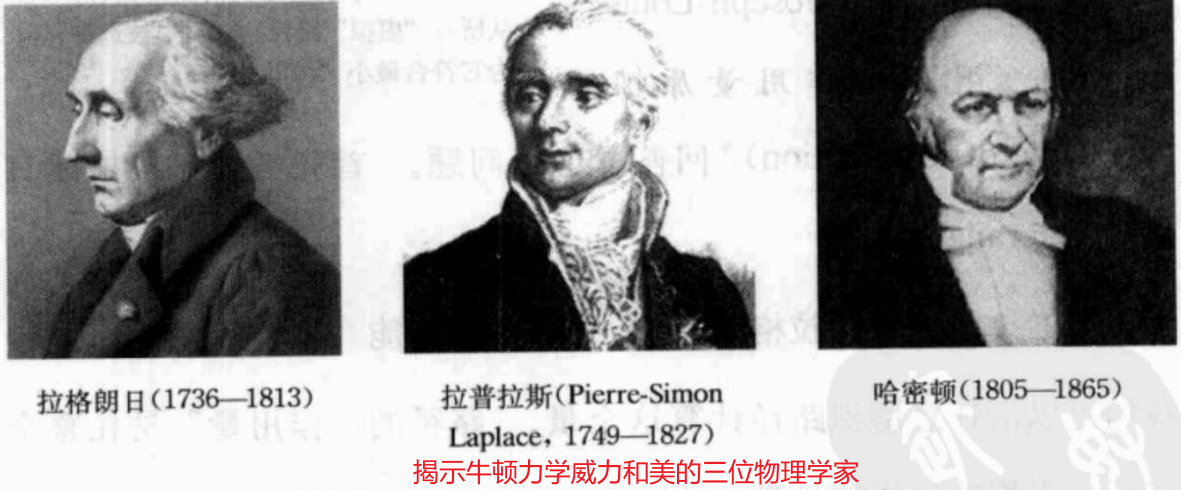## 理论力学

Hamiltonian Mechanics Sequence

### Lagrangian and Hamiltonian Mechanics#### Newton's Approach#### Hamilton's Approach

To specify what the pendulum is doing at any given instant, 我们需要知道位置和速度，或者等价地知道位置和动量$$(\theta_{0}, p_{0})$$，通过初始条件就可以借助哈密顿量$$(\theta(t), p(t))$$求解算出后面任意时刻的运动状态

$$\theta-p$$相平面中的曲线$$(\theta(t), p(t))$$叫作a flow on the phase space。这些flows很特别，they won't travel along any old curve in the plane。因为单摆的能量守恒，如果我们在某个flow curve的任意时刻去evaluate the Hamiltonian，we're guaranteed to always get the same number— $$H$$ is a constant of the flow. 这意味着不管初始的哈密顿量$$H_0$$是多少，the flow will be trapped on the curve in the $$\theta-p$$ plane where the energy is $$H(\theta, p)=H_{0}$$。参考动画This is only the tip of the iceberg for Lagrangian and Hamiltonian mechanics. Not only are these fascinating and extremely useful approaches to classical mechanics, they are fundamental to the way we think about quantum mechanics. For example, functions on phase space in classical mechanics turn into operators on the space of quantum states in quantum mechanics. If you know the state $$|\psi\rangle$$ of a quantum system at $$t=0$$, the Schrödinger equation says that the state at a later time $$t$$ will be $$e^{-\frac{i}{\hbar} H t}|\psi\rangle$$, where $$H$$ is the operator versior of the classical Hamiltonian function. The Lagrangian, meanwhile, appears in the path-integral (路径积分) formulation of quantum mechanics.

### Principle of Least Action

In terms of the potential energy then, we can write the equation of motion as$$m \ddot{x}=-\frac{\mathrm{d} U}{\mathrm{~d} x}$$动能$$K=\displaystyle\frac{1}{2} m \dot{x}^{2}$$，总能量$$E=K+U$$。但是现在我们想写出二者的difference $$K-U$$  $$L=\frac{1}{2} m \dot{x}^{2}-U(x)$$This combination is called the Lagrangian function, and right now it might look like it's coming out of left field, but let's see where it leads us. For any path $$x(t)$$ between the particle's starting position $$x\left(t_{1}\right)=x_{1}$$ and its ending position $$x\left(t_{2}\right)=x_{2}$$, define a number $$S$$ by integrating the Lagrangian along the curve: $$S=\int_{t_{1}}^{t_{2}} \mathrm{~d} t\left(\frac{1}{2} m \dot{x}(t)^{2}-U(x(t))\right)$$$$S$$ is called the action of the path. So far $$x(t)$$ can be any curve connecting the two given endpoints. We want to use the action to figure out the actual trajectory that the particle will follow.

Claim: 粒子的轨迹就是那个action $$S$$最小的那个。因此这叫作principle of least action (最小作用量原理)。注意可能求解出的是一个saddle point，但是这里我们只focus on the typical case。